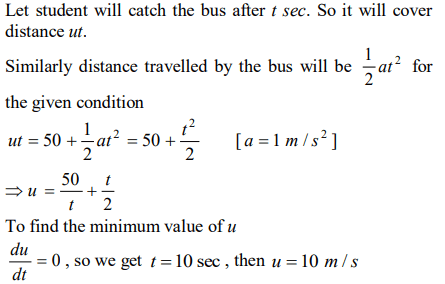## Motion in a Straight Line Questions and Answers Part-10

1. The velocity of a bullet is reduced from 200m/s to 100m/s while travelling through a wooden block of thickness 10cm. The retardation, assuming it to be uniform, will be
a) $10\times 10^{4}m\diagup s^{2}$
b) $12\times 10^{4}m\diagup s^{2}$
c) $13.5\times 10^{4}m\diagup s^{2}$
d) $15\times 10^{4}m\diagup s^{2}$

Explanation:2. A body of 5 kg is moving with a velocity of 20 m/s. If a force of 100N is applied on it for 10s in the same direction as its velocity, what will now be the velocity of the body
a) 200 m/s
b) 220 m/s
c) 240 m/s
d) 260 m/s

Explanation:3. A particle starts from rest, accelerates at $2 m\diagup s^{2}$  for 10s and then goes for constant speed for 30s and then decelerates at $4 m\diagup s^{2}$  till it stops. What is the distance travelled by it
a) 750 m
b) 800 m
c) 700 m
d) 850 m

Explanation:4. The engine of a motorcycle can produce a maximum acceleration $5 m\diagup s^{2}$. Its brakes can produce a maximum retardation 10 m/s2. What is the minimum time in which it can cover a distance of 1.5 km
a) 30 sec
b) 15 sec
c) 10 sec
d) 5 sec

Explanation:5. The path of a particle moving under the influence of a force fixed in magnitude and direction is
a) Straight line
b) Circle
c) Parabola
d) Ellipse

Explanation: Straight line

6. A car, moving with a speed of 50 km/hr, can be stopped by brakes after at least 6m. If the same car is moving at a speed of 100 km/hr, the minimum stopping distance is
a) 6m
b) 12m
c) 18m
d) 24m

Explanation:7. A student is standing at a distance of 50metres from the bus. As soon as the bus begins its motion with an acceleration of $1 m\diagup s^{2}$, the student starts running towards the bus with a uniform velocity u . Assuming the motion to be along a straight road, the minimum value of u , so that the student is able to catch the bus is
a) $5 m s^{-1}$
b) $8 m s^{-1}$
c) $10 m s^{-1}$
d) $12 m s^{-1}$

Explanation:8. A body A moves with a uniform acceleration a and zero initial velocity. Another body B, starts from the same point moves in the same direction with a constant velocity v . The two bodies meet after a time t . The value of t is
a) $\frac{2v}{a}$
b) $\frac{v}{a}$
c) $\frac{v}{2a}$
d) $\sqrt{\frac{v}{2a}}$

Explanation:9. A particle moves along X-axis in such a way that its coordinate X varies with time t according to the equation $x=\left(2-5t+6t^{2}\right)m$    . The initial velocity of the particle is
a) $-5m \diagup s$
b) $6m \diagup s$
c) $-3m \diagup s$
d) $3m \diagup s$a) $\frac{aT}{4}$
b) $\frac{3aT}{2}$
c) $\frac{aT}{2}$